JEE  >  WBJEE Phy & Chem Sample Paper

# WBJEE Phy & Chem Sample Paper

Test Description

## 80 Questions MCQ Test WBJEE Sample Papers, Section Wise & Full Mock Tests | WBJEE Phy & Chem Sample Paper

WBJEE Phy & Chem Sample Paper for JEE 2022 is part of WBJEE Sample Papers, Section Wise & Full Mock Tests preparation. The WBJEE Phy & Chem Sample Paper questions and answers have been prepared according to the JEE exam syllabus.The WBJEE Phy & Chem Sample Paper MCQs are made for JEE 2022 Exam. Find important definitions, questions, notes, meanings, examples, exercises, MCQs and online tests for WBJEE Phy & Chem Sample Paper below.
Solutions of WBJEE Phy & Chem Sample Paper questions in English are available as part of our WBJEE Sample Papers, Section Wise & Full Mock Tests for JEE & WBJEE Phy & Chem Sample Paper solutions in Hindi for WBJEE Sample Papers, Section Wise & Full Mock Tests course. Download more important topics, notes, lectures and mock test series for JEE Exam by signing up for free. Attempt WBJEE Phy & Chem Sample Paper | 80 questions in 120 minutes | Mock test for JEE preparation | Free important questions MCQ to study WBJEE Sample Papers, Section Wise & Full Mock Tests for JEE Exam | Download free PDF with solutions
 1 Crore+ students have signed up on EduRev. Have you?
WBJEE Phy & Chem Sample Paper - Question 1

### One Kg of copper is drawn into a wire of 1mm diameter and a wire of 2 mm diameter. The resistance of the two wires will be in the ratio

Detailed Solution for WBJEE Phy & Chem Sample Paper - Question 1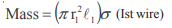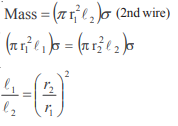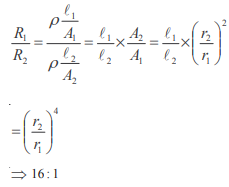WBJEE Phy & Chem Sample Paper - Question 2

### An electrical cable having a resistance of 0.2 : delivers 10kw at 200V D.C. to a factory. What is the efficiency of transmission?

Detailed Solution for WBJEE Phy & Chem Sample Paper - Question 2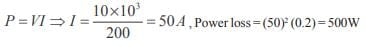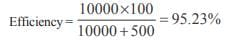WBJEE Phy & Chem Sample Paper - Question 3

### A wire of resistance 5Ω is drawn out so that its new length is 3 times its original length.What is the reistance of the new wire?

Detailed Solution for WBJEE Phy & Chem Sample Paper - Question 3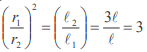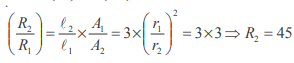WBJEE Phy & Chem Sample Paper - Question 4

Two identical cells each of emf E and internal resistance r are connected in parallel with an external resistance R. To get maximum power developed across R, the value of R is

Detailed Solution for WBJEE Phy & Chem Sample Paper - Question 4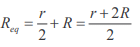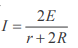For max. power consumption. I should be max. So denominator should be min. for that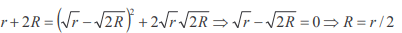WBJEE Phy & Chem Sample Paper - Question 5

To write the decimal number 37 in binary, how many binary digits are required?

Detailed Solution for WBJEE Phy & Chem Sample Paper - Question 5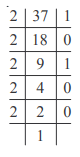(100101) ⇒ 6 digits

WBJEE Phy & Chem Sample Paper - Question 6

A junction diode has a resistance of 25Ω when forward biased and 2500Ω when reverse biased. The current in the diode, for the arrangement shown will be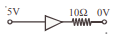Detailed Solution for WBJEE Phy & Chem Sample Paper - Question 6

Req = 25 + 10 = 35Ω
Because diode is forward biased. So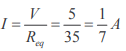WBJEE Phy & Chem Sample Paper - Question 7

If the electron in a hydrogen atom jumps from an orbit with level n1 = 2 to an orbit with level n2 = 1 the emitted radiation has a wavelength given by

Detailed Solution for WBJEE Phy & Chem Sample Paper - Question 7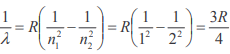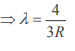WBJEE Phy & Chem Sample Paper - Question 8

What is the particle x in the following nuclear reaction: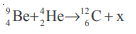Detailed Solution for WBJEE Phy & Chem Sample Paper - Question 8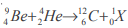Hence X represents neutron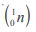WBJEE Phy & Chem Sample Paper - Question 9

An alternating current of rms value 10 A is passed through a 12Ω resistor. The maximum potential difference across the resistor is

Detailed Solution for WBJEE Phy & Chem Sample Paper - Question 9

Irms = 10A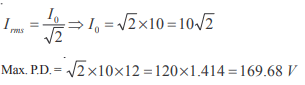WBJEE Phy & Chem Sample Paper - Question 10

Which of the following relation represent Biot-Savart’s law?

Detailed Solution for WBJEE Phy & Chem Sample Paper - Question 10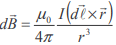WBJEE Phy & Chem Sample Paper - Question 11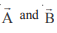are two vectors given by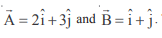The magnitude of the component of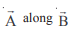is

Detailed Solution for WBJEE Phy & Chem Sample Paper - Question 11

Magnitude of components of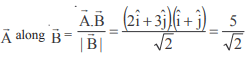WBJEE Phy & Chem Sample Paper - Question 12

Given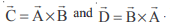What is the angle between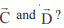Detailed Solution for WBJEE Phy & Chem Sample Paper - Question 12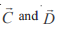are antiparellel since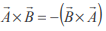WBJEE Phy & Chem Sample Paper - Question 13

The acceleration ‘a’ (in ms–2) of a body, starting from rest varies with time t (in s) following the equation a = 3t + 4 The velocity of the body at time t = 2s will be

Detailed Solution for WBJEE Phy & Chem Sample Paper - Question 13

a = 3t + 4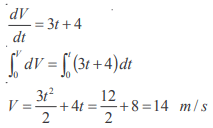WBJEE Phy & Chem Sample Paper - Question 14

Figure below shows the distance-time graph of the motion of a car. If follows from the graph that the car is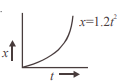Detailed Solution for WBJEE Phy & Chem Sample Paper - Question 14

Slope is increasing with constant rate. i.e motion is uniformaly accelerated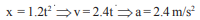WBJEE Phy & Chem Sample Paper - Question 15

Two particles have masses m & 4m and their kinetic energies are in the ratio 2: 1. What is the ratio of their linear momenta ?

Detailed Solution for WBJEE Phy & Chem Sample Paper - Question 15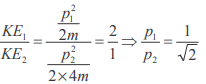WBJEE Phy & Chem Sample Paper - Question 16

The force F acting on a particle moving in a straight line is shown below. What is the work done by the force on the particle in the 1st meter of the trajectory ?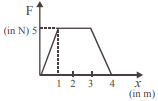Detailed Solution for WBJEE Phy & Chem Sample Paper - Question 16

Work done in 1 meter = area of shaded curve = 1/2 × 1×5 =2.5 J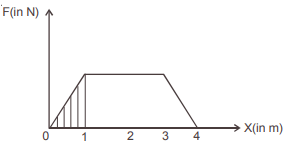WBJEE Phy & Chem Sample Paper - Question 17

If the kinetic energy of a body changes by 20% then its momentum would change by –

Detailed Solution for WBJEE Phy & Chem Sample Paper - Question 17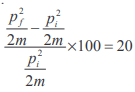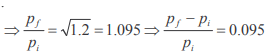Therefore % increase = 9.5%

WBJEE Phy & Chem Sample Paper - Question 18

A bullet is fired with a velocity u making an angle of 60° with the horizontal plane. The horizontal component o the velocity of the bullet when it reaches the maximum height is

Detailed Solution for WBJEE Phy & Chem Sample Paper - Question 18

Horizontal velocity would be constant so the value of velocity at the highest point will be u/2

WBJEE Phy & Chem Sample Paper - Question 19

A particle is projected at 60° to the horizontal with a kinetic energy K. The kinetic energy at the highest point is

Detailed Solution for WBJEE Phy & Chem Sample Paper - Question 19

At highest point kinetic energy = 1/2m (v cos 60°)2 = 1/4 × 1/2m v2 = K/4

WBJEE Phy & Chem Sample Paper - Question 20

The poisson’s ratio of a material is 0.5. If a force is applied to a wire of this material, there is a decrease in the cross-sectional area by 4%. The percentage increase in the length is :

Detailed Solution for WBJEE Phy & Chem Sample Paper - Question 20

Poisson ratio = 0.5
Therefore density is constant hence change in volume is zero we haveThat is 4%

WBJEE Phy & Chem Sample Paper - Question 21

Two spheres of equal masses but radii r1 and r2 are allowed to fall in a liquid of infinite column. The ratio of their terminal velocities is

Detailed Solution for WBJEE Phy & Chem Sample Paper - Question 21

We have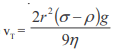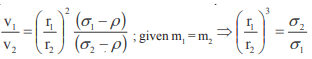WBJEE Phy & Chem Sample Paper - Question 22

Two massless springs of force constants K1 and K2 are joined end to end. The resultant force constant K of the system is

Detailed Solution for WBJEE Phy & Chem Sample Paper - Question 22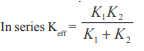WBJEE Phy & Chem Sample Paper - Question 23

A spring of force constant k is cut into two equal halves. The force constant of each half is

Detailed Solution for WBJEE Phy & Chem Sample Paper - Question 23

As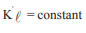K' =  2K

WBJEE Phy & Chem Sample Paper - Question 24

Two rods of equal length and diameter have thermal conductivities 3 and 4 units respectively. If they are joined in series, the thermal conductivity of the combination would be

Detailed Solution for WBJEE Phy & Chem Sample Paper - Question 24

In series R = R1 + R2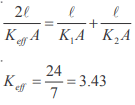WBJEE Phy & Chem Sample Paper - Question 25

19 g of water at 30° C and 5 g of ice at – 20° C are mixed together in a calorimeter. What is the final temperature of the mixture? Given specific heat of ice = 0.5 cal g–1(°C)–1 and latent heat of fusion of ice = 80 cal g–1

Detailed Solution for WBJEE Phy & Chem Sample Paper - Question 25

5 × .5 × 20 + 5 × 80 + 5t = 19 × 1 × (30 – t)
t = 5°C

WBJEE Phy & Chem Sample Paper - Question 26

. It is difficult to cook rice in an open vessel by boiling it at high altitudes because of

Detailed Solution for WBJEE Phy & Chem Sample Paper - Question 26

At high altitude pressure is low and boiling point also low

WBJEE Phy & Chem Sample Paper - Question 27

The height of a waterfall is 50 m. If g = 9.8 ms–2 the difference between the temperature at the top and the bottom of the waterfall is:

Detailed Solution for WBJEE Phy & Chem Sample Paper - Question 27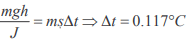WBJEE Phy & Chem Sample Paper - Question 28

The distance between an object and a divergent lens is m times the focal length of the lens. The linear magnification produced by the lens is

Detailed Solution for WBJEE Phy & Chem Sample Paper - Question 28

u = – mf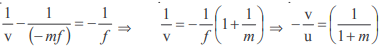WBJEE Phy & Chem Sample Paper - Question 29

A 2.0 cm object is placed 15 cm in front of a concave mirror of focal length 10 cm. What is the size and nature of the image?

Detailed Solution for WBJEE Phy & Chem Sample Paper - Question 29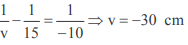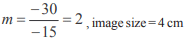WBJEE Phy & Chem Sample Paper - Question 30

A beam of monochromatic blue light of wavelength 4200 Å in air travels in water of refractive index 4/3. Its wavelength in water will be:

Detailed Solution for WBJEE Phy & Chem Sample Paper - Question 30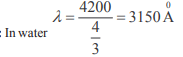WBJEE Phy & Chem Sample Paper - Question 31

Two identical light waves, propagating in the same direction, have a phase difference G. After they superpose the intensity of the resulting wave will be proportional to

Detailed Solution for WBJEE Phy & Chem Sample Paper - Question 31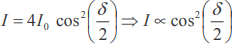WBJEE Phy & Chem Sample Paper - Question 32

The equation of state for n moles of an ideal gas is PV = nRT, where R is a constant. The SI unit for R is

Detailed Solution for WBJEE Phy & Chem Sample Paper - Question 32

JK–1 mol–1

WBJEE Phy & Chem Sample Paper - Question 33

At a certain place, the horizontal component of earth’s magnetic field is √3 times the vertical component. The angle of dip at that place is

Detailed Solution for WBJEE Phy & Chem Sample Paper - Question 33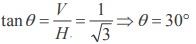WBJEE Phy & Chem Sample Paper - Question 34

The number of electron in 2 coulomb of charge is

Detailed Solution for WBJEE Phy & Chem Sample Paper - Question 34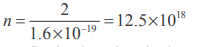WBJEE Phy & Chem Sample Paper - Question 35

The current flowing through a wire depends on time as I = 3t2 +2t + 5. The charge flowing through the cross section of the wire in time from t = 0 to t = 2 sec. is

Detailed Solution for WBJEE Phy & Chem Sample Paper - Question 35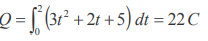WBJEE Phy & Chem Sample Paper - Question 36

If the charge on a capacitor is increased by 2 coulomb, the energy stored in it increases by 21%. The original charge on the capacitor is

Detailed Solution for WBJEE Phy & Chem Sample Paper - Question 36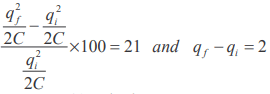solving we get qi = 20 coulomb

WBJEE Phy & Chem Sample Paper - Question 37

The work done in carrying a charge Q once around a circle of radius r about a charge q at the centre is

Detailed Solution for WBJEE Phy & Chem Sample Paper - Question 37

Work done by conservative force in a round trip is zero

WBJEE Phy & Chem Sample Paper - Question 38

Four capacitors of equal capacitance have an equivalent capacitance C1 when connected in series and an equivalent capacitance C2 when connected in parallel. The ratio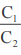is:

Detailed Solution for WBJEE Phy & Chem Sample Paper - Question 38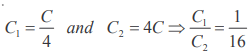WBJEE Phy & Chem Sample Paper - Question 39

Magnetic field intensity H at the centre of a circular loop of radius r carrying current I e.m.u is

Detailed Solution for WBJEE Phy & Chem Sample Paper - Question 39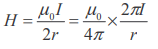In e.m.u system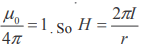WBJEE Phy & Chem Sample Paper - Question 40

Which of the following materials is the best conductor of electricity?

WBJEE Phy & Chem Sample Paper - Question 41

Which statement is incorrect

Detailed Solution for WBJEE Phy & Chem Sample Paper - Question 41

Phenol does not liberate CO2 from Na2 CO3 solution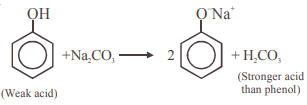Note : Strong acid is not formed by weak acid

WBJEE Phy & Chem Sample Paper - Question 42

In which of the following reactions new carbon-carbon bond is not formed :

Detailed Solution for WBJEE Phy & Chem Sample Paper - Question 42

In cannizaro’s reaction no new C–C bond is formed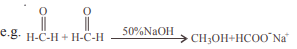WBJEE Phy & Chem Sample Paper - Question 43

A compound is formed by substitution of two chlorine for two hydrogens in propane. The number of possible isomeric compounds is

Detailed Solution for WBJEE Phy & Chem Sample Paper - Question 43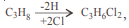following isomers of C3H6 Cl2 is possible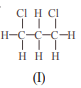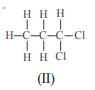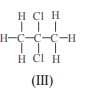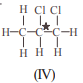Due to presence of chiral carbon compound (IV) is optically active and forms an enantiomer. So total no of isomers =5

WBJEE Phy & Chem Sample Paper - Question 44

Which one of the following is called a carbylamine?

WBJEE Phy & Chem Sample Paper - Question 45

For making distinction between 2-pentanone and 3-pentanone the reagent to be employed is

Detailed Solution for WBJEE Phy & Chem Sample Paper - Question 45

In 2-pentanone ie.,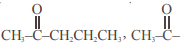group is present due to which it can show iodoform test. i.e.,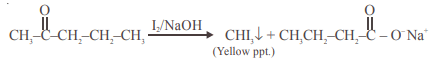WBJEE Phy & Chem Sample Paper - Question 46

Which one of the following formulae does not represent an organic compound?

Detailed Solution for WBJEE Phy & Chem Sample Paper - Question 46

Unsaturation factor = 0, 1, 1, 0.5 Hence (D)

WBJEE Phy & Chem Sample Paper - Question 47

The catalyst used for olefin polymerization is

Detailed Solution for WBJEE Phy & Chem Sample Paper - Question 47

TiCl3 + (C2H5 )3 Al

WBJEE Phy & Chem Sample Paper - Question 48

The oxidant which is used as an antiseptic is :

WBJEE Phy & Chem Sample Paper - Question 49

Which of the following contributes to the double helical structure of DNA

WBJEE Phy & Chem Sample Paper - Question 50

The monomer used to produce orlon is

Detailed Solution for WBJEE Phy & Chem Sample Paper - Question 50

Orlon or PAN
Monomer ⇒ CH2 =CH–CN

WBJEE Phy & Chem Sample Paper - Question 51

1 mole of photon, each of frequency 2500 S-1, would have approximately a total energy of :

Detailed Solution for WBJEE Phy & Chem Sample Paper - Question 51

Total Energy = Nhv = 6.022 × 1023 × 6.626 × 10–34 J.S. × 2500 s–1 = 9.9 erg ≈ 10 erg
In (A) option, it should be 10 erg instead of 1 erg.

WBJEE Phy & Chem Sample Paper - Question 52

If nt number of radioatoms are present at time t, the following expression will be a constant :

Detailed Solution for WBJEE Phy & Chem Sample Paper - Question 52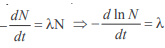Hence (C)

WBJEE Phy & Chem Sample Paper - Question 53

The following graph shows how T1/2 (half-life) of a reactant R changes with the initial reactant concentration a0 .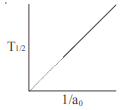The order of the reaction will be:

Detailed Solution for WBJEE Phy & Chem Sample Paper - Question 53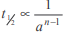Hence (C)

WBJEE Phy & Chem Sample Paper - Question 54

The second law of thermodynamics says that in a cyclic process :

Detailed Solution for WBJEE Phy & Chem Sample Paper - Question 54

Because 0 K temperature is unattainable

WBJEE Phy & Chem Sample Paper - Question 55

The equilibrium constant (K) of a reaction may be written as :

Detailed Solution for WBJEE Phy & Chem Sample Paper - Question 55

ΔGº = –RT lnK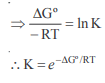WBJEE Phy & Chem Sample Paper - Question 56

For the reaction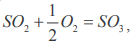if we write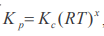then x becomes

Detailed Solution for WBJEE Phy & Chem Sample Paper - Question 56

KP = KC(RT)x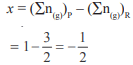WBJEE Phy & Chem Sample Paper - Question 57

If it is assumed that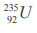decays only by emitting α and  β particles, the possible product of the decay is :

Detailed Solution for WBJEE Phy & Chem Sample Paper - Question 57

New mass no. = 235 – 2 × 4 = 227
New at. no. = 92 – 2 × 2 + 1 = 92 – 4 + 1 = 89

WBJEE Phy & Chem Sample Paper - Question 58

The time taken for 10% completion of a first order reactin is 20 mins. Then, for 19% completion, the reaction will take

Detailed Solution for WBJEE Phy & Chem Sample Paper - Question 58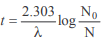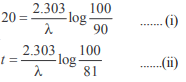equation (i) / (ii)
∴ t = 40 min.

WBJEE Phy & Chem Sample Paper - Question 59

Which of the following will decrease the pH of a 50 ml solution of 0.01 M HCl?

Detailed Solution for WBJEE Phy & Chem Sample Paper - Question 59

50 ml 0.01 M ≡ 50 × 0.01 = 0.5
millimole 5 ml 1 (M) ≡ 5 × 1 = 5 millimole
Total millimoles = 5.5 millimole
Total volume = 55 ml.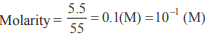pH = 1

WBJEE Phy & Chem Sample Paper - Question 60

Equal volumes of molar hydrochloric acid and sulphuric acid are neutralised by dilute NaOH solution and x kcal and y kcal of heat are liberated respectively. Which of the following is true?

Detailed Solution for WBJEE Phy & Chem Sample Paper - Question 60

Enthalpy of 1 g equivalent of strong acid and 1 g equivalent strong base = 13.7 kcal
Equal volume contains double eq. of H2SO4 than HCl

WBJEE Phy & Chem Sample Paper - Question 61

Hybridisation of central atom in NF3 is

Detailed Solution for WBJEE Phy & Chem Sample Paper - Question 61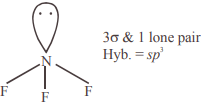WBJEE Phy & Chem Sample Paper - Question 62

Of the following compounds the most acidic is

Detailed Solution for WBJEE Phy & Chem Sample Paper - Question 62

In a group as we go downwards, the oxide basic character increases hence maximum acidic oxide is P2O5

WBJEE Phy & Chem Sample Paper - Question 63

The half-life of a radioactive element is 10 hours. How much will be left after 4 hours in 1 g atom sample?

Detailed Solution for WBJEE Phy & Chem Sample Paper - Question 63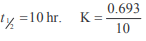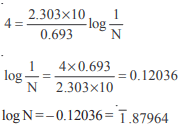N = 7.575 × 10–1 g atoms
∴ No. of atoms = 7.575 × 10–1 × 6.023 × 1023 atoms = 4.56 × 1023 atoms

WBJEE Phy & Chem Sample Paper - Question 64

For the Paschen series the values of n1 and n2 in the expression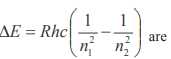Detailed Solution for WBJEE Phy & Chem Sample Paper - Question 64

In Paschen series electron shifting to third shell i.e., n1 = 3 to n2 = 4, 5, 6,

WBJEE Phy & Chem Sample Paper - Question 65

Under which of the following condition is the relation ΔH = ΔE + PΔV valid for a closed system?

Detailed Solution for WBJEE Phy & Chem Sample Paper - Question 65

This is applicable when pressure remains constant

WBJEE Phy & Chem Sample Paper - Question 66

An organic compound made of C, H and N contains 20% nitrogen. Its molecular weight is :

Detailed Solution for WBJEE Phy & Chem Sample Paper - Question 66

Nitrogen at. wt. = 14 in a molecule minimum one atom of N is present
i.e.,    20% ≡ 14             Molecular weight = 70
100% ≡ 14 × 5 = 70

WBJEE Phy & Chem Sample Paper - Question 67

In Cu-ammonia complex, the state of hybridization of Cu+2 is

Detailed Solution for WBJEE Phy & Chem Sample Paper - Question 67

In   [Cu(NH3)4]+
Cu+2 is in a state of dsp2 hybridization and shape of the complex is square planar. (One eis excited from 3d to 4p during complex formation)

WBJEE Phy & Chem Sample Paper - Question 68

The reaction that takes place when Cl2 gas is passed through conc. NaOH solution is :

Detailed Solution for WBJEE Phy & Chem Sample Paper - Question 68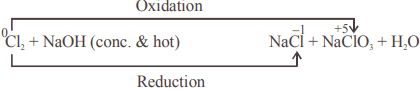Hence the reaction is disproportionation

WBJEE Phy & Chem Sample Paper - Question 69

“Electron” is an alloy of

Detailed Solution for WBJEE Phy & Chem Sample Paper - Question 69

Electron is an alloy of Mg(95%) + Zn(4.5%) and Cu(0.5%)

WBJEE Phy & Chem Sample Paper - Question 70

Blackened oil painting can be restored into original form by the action of :

Detailed Solution for WBJEE Phy & Chem Sample Paper - Question 70

Blackening of oil painting is due to PbS which is oxidised by H2O2 to form white PbSO4
PbS + H2O2 → PbSO4 + H2O
(Black)                (white)

WBJEE Phy & Chem Sample Paper - Question 71

Of the following acids the one which has the capability to form complex compound and also possesses oxidizing and reducing properties is :

Detailed Solution for WBJEE Phy & Chem Sample Paper - Question 71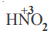Here oxidation state of N lies between –3 to +5

WBJEE Phy & Chem Sample Paper - Question 72

Atoms in a P4 molecule of white phosphorus are arranged regularly in the following way :

Detailed Solution for WBJEE Phy & Chem Sample Paper - Question 72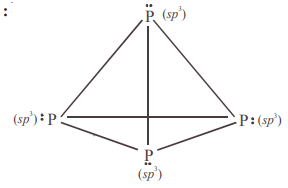WBJEE Phy & Chem Sample Paper - Question 73

Which of the following statements is not correct

Detailed Solution for WBJEE Phy & Chem Sample Paper - Question 73

Silicon exist in nature in combined state as SiO2

WBJEE Phy & Chem Sample Paper - Question 74

4. In aluminium extraction by the Bayer process, alumina is extracted from bauxite by sodium hydroxide at high temperature and pressures :​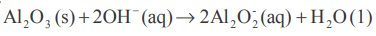Solid impurities such as Fe2O3 and SiO2 are removed and then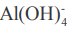is reprecipitated :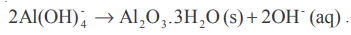In the industrial world :

WBJEE Phy & Chem Sample Paper - Question 75

The addition of HBr to 2-pentene gives

Detailed Solution for WBJEE Phy & Chem Sample Paper - Question 75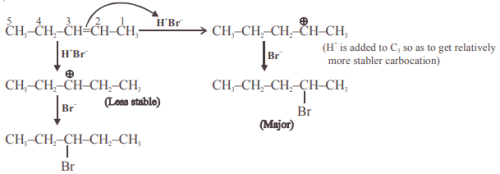WBJEE Phy & Chem Sample Paper - Question 76

Ethelene can be separated from acetylene by passing the mixture through :

Detailed Solution for WBJEE Phy & Chem Sample Paper - Question 76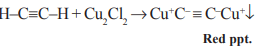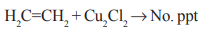WBJEE Phy & Chem Sample Paper - Question 77

Reaction of R OH with R' MgX produces :

Detailed Solution for WBJEE Phy & Chem Sample Paper - Question 77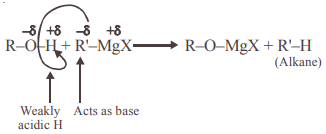WBJEE Phy & Chem Sample Paper - Question 78

In the compound HC ≡ C - CH = CH2 the hybridization of C–2 and C–3 carbons are respectively :

Detailed Solution for WBJEE Phy & Chem Sample Paper - Question 78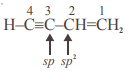(Double bond is preferred)

WBJEE Phy & Chem Sample Paper - Question 79

The two structures written below represent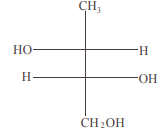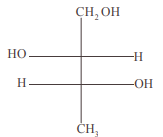Detailed Solution for WBJEE Phy & Chem Sample Paper - Question 79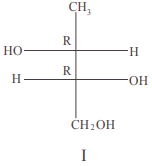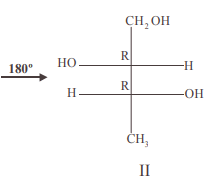I & II are same Fischer projection because 180º rotation doesn't change configuration

WBJEE Phy & Chem Sample Paper - Question 80

Which of the following carbocations will be most stable ?

Detailed Solution for WBJEE Phy & Chem Sample Paper - Question 80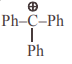(Highly resonance stabilized)

## WBJEE Sample Papers, Section Wise & Full Mock Tests

4 videos|10 docs|54 tests
 Use Code STAYHOME200 and get INR 200 additional OFF Use Coupon Code
Information about WBJEE Phy & Chem Sample Paper Page
In this test you can find the Exam questions for WBJEE Phy & Chem Sample Paper solved & explained in the simplest way possible. Besides giving Questions and answers for WBJEE Phy & Chem Sample Paper , EduRev gives you an ample number of Online tests for practice

## WBJEE Sample Papers, Section Wise & Full Mock Tests

4 videos|10 docs|54 tests